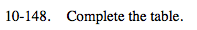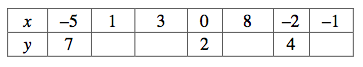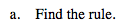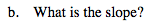### Home > MC2 > Chapter 10 > Lesson 10.2.6 > Problem10-148

10-148.Do you see any patterns?
Notice the difference in the y-value from x = 0 to x = −2.

Also notice that as the x-value decreases, the y-value increases, and as the x-value increases, the y-value decreases.

y = −x + 2$\text{Slope = \frac{rise}{run}}$

−1

Complete the table in the eTool below.
Click the link at right for the full version of the eTool: MC2 10-146 HW eTool